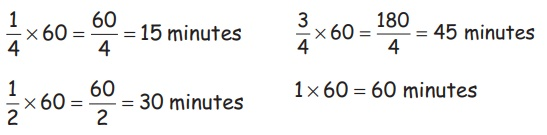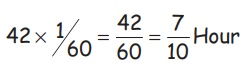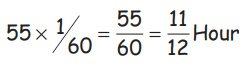Home | | Maths 5th Std | Use fractions in the context of units of length, time and money

# Use fractions in the context of units of length, time and money

Use fractions in the context of units of length, time and money

Use fractions in the context of units of length, time and money

Length (In fractions)

Example

2 Km 500 m = 2 ½ Km

1 Km 250 m = 1 ¼ Km

7 Km 750 m = 7 ¾ Km

8 Km 500 m = 8 ½ Km

Let us know

1 Km = 1000 m

½ Km = 500 m

¾ Km = 750 m

¼ Km = 250 m

Time (In fractions)

15 minutes = ¼ Hour

30 minutes = ½ Hour

45 minutes = ¾ Hour

60 minutes = 1 Hour1/4 × 60 = 60/4 = 15 minutes

1/2 × 60 = 60/2 = 30 minutes

3/4 × 60 = 180/4 = 45 minutes

1 × 60 = 60minutes

Let us know

60 minutes = 1 Hours

Half part of 60 minutes = 30 minutes

Quarter part of 60 minutes = 15 minutes

There Quarter part of 60 minutes = 45 minutes

Examples

1. Convert into minutes

a) 2 Hour = 2 × 60 = 120 minutes 1 Hour = 60 minutes

b) 3 Hour 20 minutes = (3 × 60) + 20 = 200 minutes

c) 4 Hour = 4 × 60 = 240 minutes

d) 5 Hour 15 minutes = (5 × 60) + 15 = 300 + 15 = 315 minutes

2. Convert in to hours

a) 42 minutes42 × 1/60 = 42/60 = 7/10 Hour

b) 55 minutes55 × 1/60 = 55/60 = 11/12 Hour

Money [In fractions]

Example

In 1

¼ part of ₹ 1 = 25 paise Quarter part of ₹ 1 = 25 paise

½ part of ₹ 1 = 50 paise Half part of ₹ 1 = 50 paise

¾ part of ₹ 1 = 75 paise Three quarter part of ₹ 1 = 75 paise

1 part of ₹ 1 = 100 paise Full part of ₹ 1 = 100 paise

Example

In 100

¼ part of ₹ 100 = ₹ 25 Quarter part of ₹100 = ₹ 25

½ part of ₹ 100 = ₹ 50 Half part of ₹100 = ₹ 50

¾ part of ₹ 100 = ₹ 75 Three quarter part of ₹100 = ₹ 75

1 part of ₹ 100 = ₹ 100 Full part of ₹100 = ₹100

Example

In 2000

¼ part of ₹2000 = ₹500 Quarter part of ₹2000 = ₹500

½ part of ₹2000 = ₹1000 Half part of ₹2000 = ₹1000

¾ part of ₹2000= ₹1500 Three quarter part of ₹2000= ₹1500

1 part of ₹2000 = ₹2000 Full part of ₹2000 = ₹2000

Tags : Interconcept | Term 2 Chapter 5 | 5th Maths , 5th Maths : Term 2 Unit 5 : Interconcept
Study Material, Lecturing Notes, Assignment, Reference, Wiki description explanation, brief detail
5th Maths : Term 2 Unit 5 : Interconcept : Use fractions in the context of units of length, time and money | Interconcept | Term 2 Chapter 5 | 5th Maths